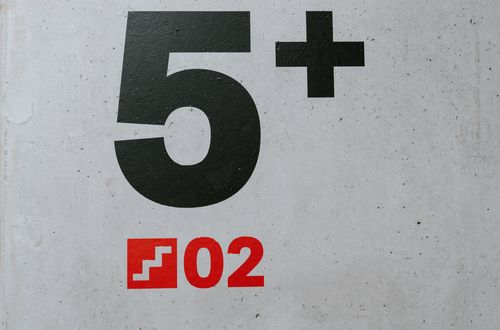# How to increase a number by a percentage

Want to find out how to increase a number by a percentage? We've provided a step-by-step guide of how to increase a number by a percentage to teach you everything you need to know.## Step 1: Add the percentage and 100

Add the percentage and 100. So, if we want to increase 75 by 20%, we add:

## Step 2: Change the percentage to a decimal form

Move two decimal places to the left of the percentage. Hence, we move two decimal places to the left of 120.

## Step 3: Multiply by the base value

Once we have the decimal form of the percentage, we multiply it with the given base value. So, for the case of our example, if we increase 75 by 20%, then the result would be 90.

## Examples of increasing a number by a percentage

Q1) What would the result be if 150 is increased by 30%?

First, we can add 100% and 30%, 100+30=130%

Once the percentages are added, we move two decimal places to the left of 130%.

130% = 1.30

Multiply the decimal by 150 to find the new value.

1.30×150 = 195

Then, the result would be 195.

Q2) Blair’s average last semester was 88 and she plans to increase this the next semester by 5%. What should she expect her minimum average be if she succeeded in increasing her grade?

We first add 100 and 5 : 100+5=105%. Next, we move two decimal places to the left of 105% .

105%=1.05

To increase 88 by 5%, we multiply 88 by 1.05. 1.05×88=92.4

This means that Blair should at least have 92.4 for her average score next semester.

#### Join today

The fastest way to practice

Unlock our complete testing platform and improve faster that ever.